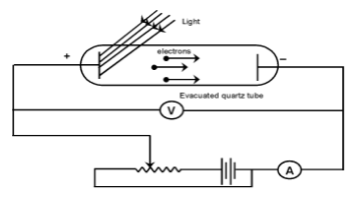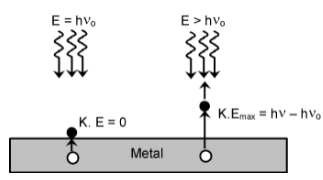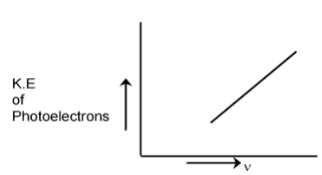# Photo Electric Effect

## Atomic Structure of Class 11

Sir J.J. Thomson, observed that when a light of certain frequency strikes the surface of a metal, electrons are ejected from the metal. This phenomenon is known as photoelectric effect  and the ejected electrons are called photoelectrons.

A few metals, which are having low ionisation energy like Cesium, show this effect under the action of visible light but many more show it under the action of more energetic ultraviolet light.An evacuated tube contains two electrodes connected to a source of variable voltage, with the metal plate whose surface is irradiated as the anode. Some of the photoelectrons that emerge from this surface have enough energy to reach the cathode despite its negative polarity, and they constitute the measured current. The slower photoelectrons are repelled before they get to the cathode. When the voltage is increased to a certain value Vo, of the order of several volts, no more photoelectrons arrive, as indicated by the current dropping to zero. This extinction voltage (or also referred as stopping potential) corresponds to the maximum photoelectron kinetic energy i.e., eVo = ½ mv2

### The experimental findings are summarised as below:

i) Electrons come out as soon as the light (of sufficient energy) strikes
the metal surface .

ii) The light of any frequency will not be able to cause ejection of electrons from a metal surface. There is a minimum frequency, called the threshold (or critical) frequency, which can just cause the ejection. This frequency varies with the nature of the metal. The higher the frequency of the light, the more energy the photoelectrons have. Blue light results in faster electrons than red light.

iii) Photoelectric current is increased with increase in intensity of light of same frequency, if emission is permitted i.e., a bright light yields more photoelectrons than a dim one of the same frequency, but the electron energies remain the same.

Light must have stream of energy particles or quanta of energy (hν). Suppose, the threshold frequency of light required to eject electrons from a metal is νo, when a photon of light of this frequency strikes a metal it imparts its entire energy (hνo) to the electron.“This energy enables the electron to break away from the atom by overcoming the attractive influence of the nucleus”. Thus each photon can eject one electron. If the frequency of light is less than νo there is no ejection of electron. If the frequency of light is higher than νo (let it be ν), the photon of this light having higher energy (hν), will impart some energy to the electron that is needed to remove it away from the atom. The excess energy would give a certain velocity (i.e, kinetic energy) to the electron.

hν = hνo + K.E

hν = hνo + ½ mv2

½ mv2 = hν–hνo

where, ν = frequency of the incident light

νo = threshold frequency

hνo is the threshold energy (or) the work function denoted by φ = hνo (minimum energy of the photon to liberate electron). It is constant for particular metal and is also equal to the ionization potential of gaseous atoms.

The kinetic energy of the photoelectrons increases linearly with the frequency of incident light. Thus, if the energy of the ejected electrons is plotted as a function of frequency, it result in a straight line whose slope is equal to Planck’s constant ‘h’ and whose
intercept is hνo.